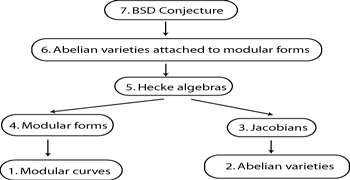# Math 252: Modular Abelian Varieties

## Instructor Information

Instructor: William Stein (Benjamin Peirce Assistant Professor of Mathematics)
Office: Science Center 515 (by the balcony)
Office Hours: Monday 2-3PM, Friday 3-4PM
Phone: 617-495-1790 (office), 617-308-0144 (mobile)
Email: [email protected]
Web page: http://modular.fas.harvard.edu/edu/Fall2003/252/

## Course Objectives

Andrew Wiles proved Fermat's Last Theorem by showing that most elliptic curves y2=x3+ax+b are "modular". In this course we will study modular elliptic curves along with their higher-dimensional generalizations, which are called "modular abelian varieties". I will not assume that you already know about modular forms and abelian varieties.

My main goal for this course is to describe how to construct an abelian variety from a modular form. I will then state the Birch and Swinnerton-Dyer Conjecture for modular abelian varieties and explain some of the main theorems and computational evidence for it, thus taking you to the forefront of current research. I will also discuss methods for computing with modular abelian varieties.

## Evaluation

There will be homework (60%) and a final project (40%), but no exams.

• Homework will consist of weekly problem sets that will be graded.
• The final project will be a paper about something related to the course. This paper could be a further exploration of examples or a proof of something not explained in the lectures.

## Textbooks

The primary textbooks for the course are Seminar on Fermat’s Last Theorem (ed. Murty) and Arithmetic Geometry (ed. Cornell and Silverman), but we will look at many other articles and books. See the references section of the web site for comments, reviews, and scans of some of the relevant material.

## Tables and Software

• Tables: You'll certainly want to look at the modular forms database and, in particular, at the modular forms explorer.
• Computations: For doing computations with modular forms and modular symbols, use the MAGMA computer algebra system. This is not a free program, but since you are in my course I can get you a free copy for Windows, Mac OS X, or Linux. I can also get you an account on the MECCAH cluster, which has MAGMA installed on it.

## Detailed OutlineI will fill in the references for the topics listed below as the semester progresses. Each bullet represents approximately one lecture.

### 1. Modular Curves

• The modular group SL2(Z): action on upper half plane, generators, relations, fundamental domain (Chapter VII of Serre's A course in arithmetic)
• Congruence subgroups: Gamma0(N), Gamma1(N), a simple method to find generators (Section III.2 of Lang's Introduction to modular forms); modular curves over C: Quotient of the upper half plane by a congruence subgroup; compactification (Section 7.1 of Diamond-Im, Section III.1 of Lang's Introduction to modular forms)
• Moduli-theoretic interpretation: Isomorphism classes of elliptic curves with level N structure (Section 7.2 of Diamond-Im); natural maps between modular curves and genus formulas
• Homology of modular curves and modular symbols; Manin symbols: finite presentation for modular symbol (Cremona, Algorithms for modular elliptic curves; Manin, Parabolic points and zeta functions of modular curves)
• Modular curves are defined over Q; equations for modular curves: Low genus, canonical embedding, Galbraith's thesis

### 2. Abelian Varieties

• Complex tori: morphisms, isogenies, endomorphisms
• Hermitian and Riemann forms
• Poincare reducibility theorem over C and semisimplicity of endomorphism algebra
• Algebraic groups and group schemes
• Theta functions and the Lefschetz embedding theorem
• Neron-Severi and picard group; polarizations of abelian varieties
• Computing intersections of complex tori
• Abelian varieties over number fields

### 3. Jacobian Varieties

• Definition; Abel-Jacobi theory (Mumford, Curves and Their Jacobians, chapter 3)
• Autoduality of Jacobians and the theta divisor
• Jacobians of modular curves, modular symbols, the cuspidal subgroup

### 4. Modular Forms

• Definitions; q-expansions, cusp forms and Eisenstein series
• Dirichlet characters and the Petersson inner product
• Multiplicity one
• Dimension formulas

### 5. Hecke Algebras

• Hecke operators as correspondences and on q-expansions
• Hecke operators on modular and Manin symbols
• Structure of Hecke algebras; Sturm bound
• Newforms and how to enumerate them

### 6. Attaching an Abelian Variety to a Modular Form

• Attaching an abelian variety to a newform
• The endomorphism ring of A over Q and the algebraic closure
• Modularity conjectures: Which abelian varieties are modular? Serre's conjectures.

### 7. The Birch and Swinnerton-Dyer Conjecture

• L-functions attached to modular forms and abelian varieties; Hecke's theorem that modular L-functions extend to the whole complex plane
• The Mordell-Weil group, heights and the regulator (sketch of proof of Mordell-Weil theorem)
• The Neron model, real volume, Manin constant, and computing L(1)/Omega
• Tamagawa numbers and component groups
• The Shafarevich-Tate group and visibility
• Statement of the Birch and Swinnerton-Dyer conjecture; results and examples
• Heegner points and the Gross-Zagier theorem
• Euler systems of Kolyvagin and Kato